# Test: Classification of Elements & Periodicity in Properties - 2

## 30 Questions MCQ Test Chemistry Class 11 | Test: Classification of Elements & Periodicity in Properties - 2

Description
Attempt Test: Classification of Elements & Periodicity in Properties - 2 | 30 questions in 60 minutes | Mock test for NEET preparation | Free important questions MCQ to study Chemistry Class 11 for NEET Exam | Download free PDF with solutions
QUESTION: 1

### Which of the following is not a Dobereiner triad -

Solution:

In Doberiner’s triad, mass of the middle element should be the average of the first and last element. In option b, Atomic massCa is not equal to the mean of atomic mass of Mg and Sr.

QUESTION: 2

### Which of the following set of elements obeyes Newland’s octave rule -

Solution:

Because Be Mg and Ca have same chemical properties.

QUESTION: 3

### Which of the following will not form crystalline structure with oppositely charged ions

Solution:

H+ ions doesn't form crystals because it hasn't any electrons .

QUESTION: 4

Which is not anomalous pair of elements in the Medeleeves periodic table -

Solution:

In Mendeleev’s periodic table, the elements are arranged in the increasing order of their atomic masses. In some places, this order has been ignored.

(a) Ar and K:  The atomic mass of argon is 40 and that of potassium is 39, But argon is placed beforepotassium in the periodic table.

(b) Co and Ni: The positions of cobalt and nickel are not in proper order. Cobalt (at. wt. = 59.9) is placed before nickel (at. wt. = 58.6).

(c) Te and I: Tellurium (at. wt. = 127.6) is placed before iodine (at. wt = 126.9).

(d) Thorium (at, wt. =232.12) is placed before protactinium (at. Wt = 231).

So, Al and Si is not the anomalous pair  of elements in the Mendeleev's periodic table.

QUESTION: 5

Elements in the same vertical group of the periodic table have same

Solution:

The periodic table also has a special name for its vertical columns. Each column is called a group. The elements in each group have the same number of electrons in the outer orbital. Those outer electrons are also called valence electrons.

QUESTION: 6

The places that were left empty by Mendeleev were, for -

Solution:

Gallium and germanium were the elements whose place were left in Mendeleev's periodic table.

QUESTION: 7

Which of the following pairs of elements do not follow octave rule -

Solution:

If the chemical elements are arranged according to increasing atomic weight, those with similar physical and chemical properties occur after each interval of seven elements. Ca,Sr do follow octave rule.

QUESTION: 8

Elements which occupied position in the lother meyer curve, on the peaks, were -

Solution:

Hydrogen, the first in the list of elements is a special case and can be considered as making up the first period all by itself. The second and third period in Meyer's table included seven elements each, and duplicated Newlands's law of octaves.

Li Be B C N O F

Na Mg Al Si P S Cl

However, the next wave had more than seven elements. The third wave had about 17 to 18 elements. This clearly showed where Newlands’ law had failed. One could not force the law of octaves to hold strictly throughout the table of elements, with seven elements in each row. After the first two periods, the length of the period had to be longer.so all the correct answer.

QUESTION: 9

Modern periodic table is based on atomic no. experiments which proved importance of at no. was -

Solution:

Moseley's work on X-ray spectra proved the significance of the atomic number.

QUESTION: 10

Atomic no. is the base of -
(i) Lother meyer curve
(ii) Newland octave rule
(iii) Modern periodic table
(v) Long form of periodic table

Solution:

Modern periodic table and long form of periodic table are same. Both are based on atomic number rather than atomic mass.

QUESTION: 11

Atomic wt. or Cl = 35.5 and of I = 127. According to doeberiner triad rule, At. wt. of Br will be -

Solution: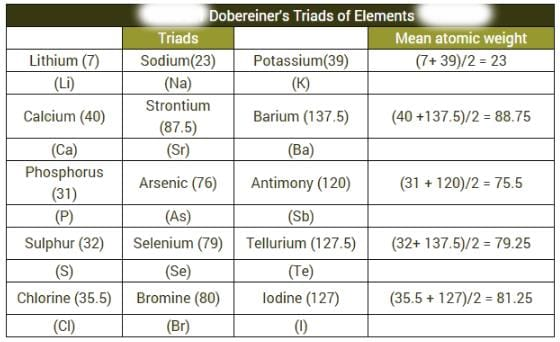QUESTION: 12

The elements of groups, 1, 2, 13, 14, 15, 16 and 17 are collectively called -

Solution:

Representative elements in modern periodic table
The first two groups on the extreme left and last six groups on the extreme right involve the filling of s- and p- orbitals, respectively, These groups represent the main groups of the periodic table and are numbered as 1, 2, 13, 14, 15, 16, 17 and 18. The elements present in these groups are known as normal elements or representative elements.

QUESTION: 13

Justification of putting H in VII A group is -

Solution:

The reason for the putting of VII A group is that the reaction of hydrogen with group 1 elements as the same as that of other halogens.

QUESTION: 14

The discovery of which of the following group of elements gave a death blow to the Newlands Law -

Solution:

The discovery of zero group of elements (inert gases) gave a death blow to the Newland's Law.

According to Newlands Law of octaves, when the elements are arranged in the increasing order of atomic weights, the properties of every eight element are similar to the first one.

QUESTION: 15

Which of the following pair of elements follows Newland’s octave rule -

Solution:

In Newland's periodic table, each row of elements consists of a total of seven elements and the eighth element falls under the first element. In the first column Lithium is the first element and sodium is the eighth element. Similarly, the eighth element after sodium is potassium. Hence from Newland's law of octaves, the elements Li, Na and K must have similar chemical and physical properties, and they do.

QUESTION: 16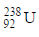(IIIB) changes to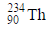by emission of α-particle. Daughter element will be in -

Solution:

The correct answer is Option A.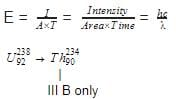Uranium-238 decays through α-particle emission to form Thorium-234

QUESTION: 17

From the list given below, elements which belongs to the same group or sub-group are -

Solution:

The correct answer is Option A.
The elements with atomic numbers 12, 20, 4, 88 belong to the same group 2.
They are Mg, Ca, Be and Ra.
They are alkaline earths and belong to the S block of the periodic table.

QUESTION: 18

The name ‘Rare earths’ is used for -

Solution:

Rare earth elements are those found in the lanthanide series of the periodic table (all 15 of them), plus scandium (Sc) and yttrium (Y). These elements are all Period 3 (that is, column 3 in the table); scandium is element 21, yttrium 39, and the lanthanides from 57 - 71.

QUESTION: 19

Z/e ratio for N3-, O2- and F- respectively will be -

Solution: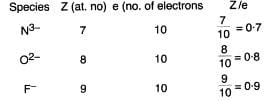QUESTION: 20

There are 10 neutrons in the nucleus of the element zM19. It belongs to -

Solution: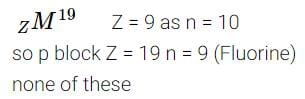QUESTION: 21

Which of the following is not an actinoid?

Solution:

Actinoids are elements with Z= 90 – 103. Therefore, Terbium (Z = 65) is not an actinoid. Terbium (Z = 65) is a lanthanoid. Tb: [Xe]4f95d06s2

QUESTION: 22

the electronic configuration of an element is 1s2 2s2 2p6 3s2 3p3. the atomic number of the element which is just below the above element in the periodic table is ________

Solution: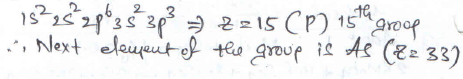QUESTION: 23

The number of elements in 5th and 6th period of periodic table are respectively -

Solution:

The first three periods containing 2, 8 and 8 elements respectively are called short periods, the next three periods containing 18,18 and 32 elements respectively are called long periods.

QUESTION: 24

Atomic number of Ag is 47. In the same group the atomic number of elements placed above and below Ag will be -

Solution:

Either learn the periodic table so the group contains copper(29) ,silver(47) and gold (79) or as you know that that copper is in fourth period so while moving from fourth to fifth period 18 is added while moving from fifth to sixth period 32 is added as the periodicity is of 2,8 ,18,18 and 32 elements.but better to learn periodic table as due to lanthanoids and actinoids there is variation in this trend.

QUESTION: 25

Atomic number 15, 33, 51 represents the following family -

Solution:

Atomic number 15, 33, 51 represents the nitrogen family. The elements with atomic numbers 15, 33 and 51 are nitrogen, arsenic and antimony respectively. They belong to group 15 (V A) of the periodic table.

QUESTION: 26

The atom having the valence shell electronic configuration 4s2 4p2 would be in -

Solution:

The atom having the valence shell electronic configuration 4s24p2 would be in Group IVA and 4 period. 'n' in electronic configuration depicts the period and the valence electrons depicts the group number.

QUESTION: 27

The number of elements know at that time when Mendeleev arranged them in the periodic table was-

Solution:

Mendeleev arranged all 63 elements; which were discovered till his time; in the order of their increasing relative atomic masses in a tabular form. It is known as Mendeleev's Periodic Table. He divided the table in eight columns and seven rows. The columns are known as groups and rows are known as periods.

QUESTION: 28

As applied to periodic table, which of the following sets include only magic numbers -

Solution:

The difference between the Nobel gases is 2, 8,8,18,18 this is a way for learning the atomic no only if we didn't remember for ex He -2 Ne -2+8=10 Ar-2+8+8 =18 kr -2+8+8+18=36 xe - 2+8+8+18+18=54 Rn - 2+8+8+18+18+32=86 in this we can learn the atomic no of elements , which helps us in electronic configuration of the atom which are above the no.30

QUESTION: 29

In the general electronic configuration -

(n - 2)f1-14 (n - 1)d0-1 ns2, if value of n = 7 the configuration will be -

Solution:

The correct answer is Option B.

General electronic configuration is given:
(n − 2)f1-14(n − 1)d01ns2 , where(n=7)
5f1-146d01ns2
The seventh period (n=7) is similar to the sixth period with the successive filling up of the 7s, 5f, 6d and 7p orbitals and includes most of the man-made radioactive elements. This period will end at the element with atomic number 118 which would belong to the noble gas family. Filling up of the 5f orbitals after actinium (Z=89) gives the 5f-inner transition series known as the Actinide Series.

QUESTION: 30

The correct order of atomic size of C, N, P, S follows the order -

Solution:

The correct order of atomic size of C, N, P, S follows the order N<C<S<P. On going from C to N, the nuclear charge increases. But the additional electron is added in the same energy level. Hence, due to shielding effect, the effective nuclear charge increase. The attraction of the nucleus for the valence electrons increases. Hence, the atomic size decreases. The atomic size of C is larger than that of N. For the same, reason, the atomic size of P is larger than that of S. The atomic size of S is larger than that of C as the electron is added to new energy level.Use Code STAYHOME200 and get INR 200 additional OFF Use Coupon Code